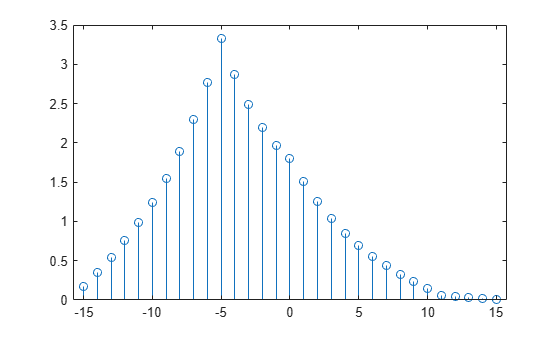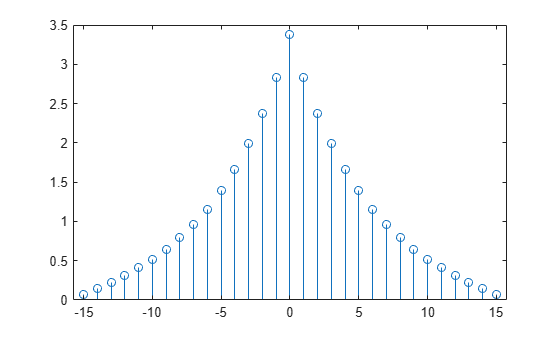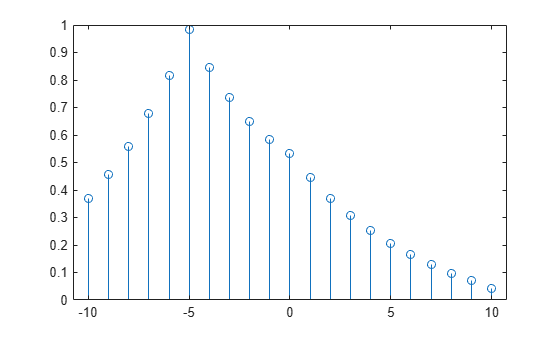xcorr

Cross-correlation

Description

example

r = xcorr(x,y) returns the cross-correlation of two discrete-time sequences. Cross-correlation measures the similarity between a vector x and shifted (lagged) copies of a vector y as a function of the lag. If x and y have different lengths, the function appends zeros to the end of the shorter vector so it has the same length as the other.

example

r = xcorr(x) returns the autocorrelation sequence of x. If x is a matrix, then r is a matrix whose columns contain the autocorrelation and cross-correlation sequences for all combinations of the columns of x.

example

r = xcorr(___,maxlag) limits the lag range from -maxlag to maxlag for either of the previous syntaxes.

example

r = xcorr(___,scaleopt) also specifies a normalization option for the cross-correlation or autocorrelation. Any option other than 'none' (the default) requires x and y to have the same length.

example

[r,lags] = xcorr(___) also returns the lags at which the correlations are computed.

Examples

collapse all

Create a vector x and a vector y that is equal to x shifted by 5 elements to the right. Compute and plot the estimated cross-correlation of x and y. The largest spike occurs at the lag value when the elements of x and y match exactly (-5).

n = 0:15;
x = 0.84.^n;
y = circshift(x,5);
[c,lags] = xcorr(x,y);
stem(lags,c)Compute and plot the estimated autocorrelation of a vector x. The largest spike occurs at zero lag, when x matches itself exactly.

n = 0:15;
x = 0.84.^n;
[c,lags] = xcorr(x);
stem(lags,c)Compute and plot the normalized cross-correlation of vectors x and y with unity peak, and specify a maximum lag of 10.

n = 0:15;
x = 0.84.^n;
y = circshift(x,5);
[c,lags] = xcorr(x,y,10,'normalized');
stem(lags,c)Input Arguments

collapse all

Input array, specified as a vector, matrix, or multidimensional array. If x is a multidimensional array, then xcorr operates column-wise across all dimensions and returns each autocorrelation and cross-correlation as the columns of a matrix.

Data Types: single | double
Complex Number Support: Yes

Input array, specified as a vector.

Data Types: single | double
Complex Number Support: Yes

Maximum lag, specified as an integer scalar. If you specify maxlag, the returned cross-correlation sequence ranges from -maxlag to maxlag. If you do not specify maxlag, the lag range equals 2N – 1, where N is the greater of the lengths of x and y.

Data Types: single | double

Normalization option, specified as one of the following.

• 'none' — Raw, unscaled cross-correlation. 'none' is the only valid option when x and y have different lengths.

• 'biased' — Biased estimate of the cross-correlation:

${\stackrel{^}{R}}_{xy,\text{biased}}\left(m\right)=\frac{1}{N}{\stackrel{^}{R}}_{xy}\left(m\right).$

• 'unbiased' — Unbiased estimate of the cross-correlation:

${\stackrel{^}{R}}_{xy,\text{unbiased}}\left(m\right)=\frac{1}{N-|m|}{\stackrel{^}{R}}_{xy}\left(m\right).$

• 'normalized' or 'coeff' — Normalizes the sequence so that the autocorrelations at zero lag equal 1:

${\stackrel{^}{R}}_{xy,\text{coeff}}\left(m\right)=\frac{1}{\sqrt{{\stackrel{^}{R}}_{xx}\left(0\right){\stackrel{^}{R}}_{yy}\left(0\right)}}{\stackrel{^}{R}}_{xy}\left(m\right).$

Output Arguments

collapse all

Cross-correlation or autocorrelation, returned as a vector or matrix.

If x is an M × N matrix, then xcorr(x) returns a (2M – 1) × N2 matrix with the autocorrelations and cross-correlations of the columns of x. If you specify maxlag, then r has size (2 × maxlag + 1) × N2.

For example, if S has three columns, $\text{S}=\left(\begin{array}{ccc}{x}_{1}& {x}_{2}& {x}_{3}\end{array}\right)$, then the result of R = xcorr(S) is organized as

$\text{R}=\left(\begin{array}{lllllllll}{R}_{{x}_{1}{x}_{1}}\hfill & {R}_{{x}_{1}{x}_{2}}\hfill & {R}_{{x}_{1}{x}_{3}}\hfill & {R}_{{x}_{2}{x}_{1}}\hfill & {R}_{{x}_{2}{x}_{2}}\hfill & {R}_{{x}_{2}{x}_{3}}\hfill & {R}_{{x}_{3}{x}_{1}}\hfill & {R}_{{x}_{3}{x}_{2}}\hfill & {R}_{{x}_{3}{x}_{3}}\hfill \end{array}\right).$

Lag indices, returned as a vector.

collapse all

Cross-Correlation and Autocorrelation

The result of xcorr can be interpreted as an estimate of the correlation between two random sequences or as the deterministic correlation between two deterministic signals.

The true cross-correlation sequence of two jointly stationary random processes, xn and yn, is given by

${R}_{xy}\left(m\right)=E\left\{{x}_{n+m}{y}_{n}^{*}\right\}=E\left\{{x}_{n}{y}_{n-m}^{*}\right\},$

where  < n < , the asterisk denotes complex conjugation, and E is the expected value operator. xcorr can only estimate the sequence because, in practice, only a finite segment of one realization of the infinite-length random process is available.

By default, xcorr computes raw correlations with no normalization:

${\stackrel{^}{R}}_{xy}\left(m\right)=\left\{\begin{array}{ll}\sum _{n=0}^{N-m-1}{x}_{n+m}{y}_{n}^{\ast },\hfill & m\ge 0,\hfill \\ {\stackrel{^}{R}}_{yx}^{*}\left(-m\right),\hfill & m<0.\hfill \end{array}$

The output vector, c, has elements given by

$\text{c}\left(\text{m}\right)={\stackrel{^}{R}}_{xy}\left(m-N\right),\text{ }\text{ }m=1,\text{\hspace{0.17em}}2,\dots ,2N-1.$

In general, the correlation function requires normalization to produce an accurate estimate. You can control the normalization of the correlation by using the input argument scaleopt.

 Buck, John R., Michael M. Daniel, and Andrew C. Singer. Computer Explorations in Signals and Systems Using MATLAB®. 2nd Edition. Upper Saddle River, NJ: Prentice Hall, 2002.

 Stoica, Petre, and Randolph Moses. Spectral Analysis of Signals. Upper Saddle River, NJ: Prentice Hall, 2005.